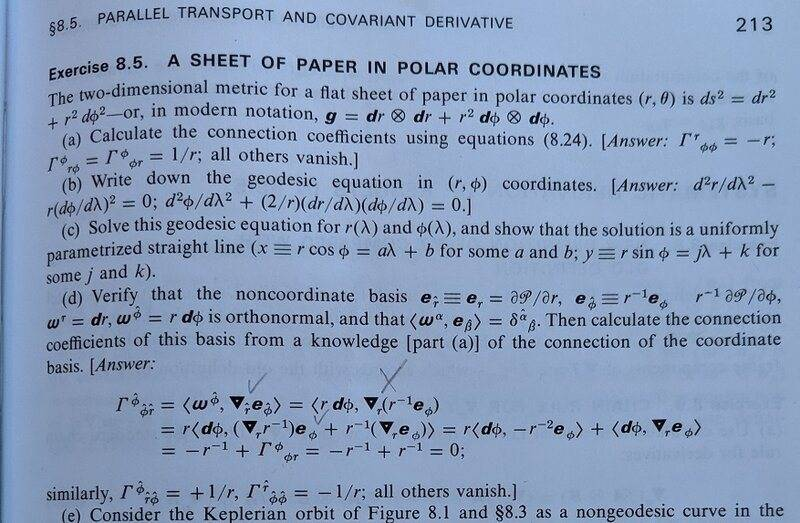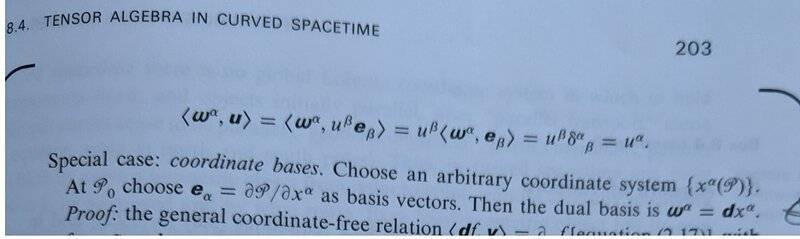# Plane polar noncoordinate basis (Latex fixed)

• LaTeX
• George Keeling
In summary: Summary:: The hat system is orthonormal because ##g\left(e_{\hat{a}},e_{\hat{b}}\right)=\delta_{\hat{a}\hat{b}}## by definition.

#### George Keeling

Gold Member
TL;DR Summary
plane polar noncoordinate basis should be orthonormal but I can't prove it
I am trying to do exercise 8.5 from Misner Thorne and Wheeler and am a bit stuck on part (d).There seem to be some typos and I would rewrite the first part of question (d) as follows

Verify that the noncoordinate basis ##{e}_{\hat{r}}\equiv{e}_r=\frac{\partial\mathcal{P}}{\partial r},\ {e}_{\hat{\phi}}\equiv r^{-1}{e}_\phi=r^{-1}\frac{\partial\mathcal{P}}{\partial\phi},\ {\omega}^{\hat{r}}={d}r,\ {\omega}^{\hat{\phi}}=r{d}\phi## is orthonormal, in other words ##<ω^\hat{\alpha},e_\hat{\beta}>=δ^\hat{\alpha}_\hat{\beta}##.

I have not followed the exact rather complicated typography. The notation ##<a,b>## is new to me and means the number of times the vector ##b## pierces the one-form ##a## (like a dot product in simple cases). One-forms are pictured as a succession of surfaces (like surfaces of equal value in a scalar field).

I wasn't sure what ##dr,d\phi## meant in this context but I found this in Box 8.4 AFrom ##\omega^\alpha=dx^\alpha## in this case it seems that we can immediately say ##{\omega}^{\hat{r}}={d}r=\ {\omega}^r,\ {\omega}^{\hat{\phi}}=r{d}\phi=r{\omega}^\phi##.

The original basis is orthogonal but not orthonormal as we can see because (I worked out)$$<\omega^r,e_r>=1,<\ \omega^r,e_\phi>=0,<\omega^\phi,e_r>=0,<\omega^\phi,e_\phi>=r$$The ##e_\phi## basis vector increases the further away from the origin one is. It looks like the ##\ e_{\hat{\phi}}## is exactly suited to cancel that.

Now it's easy to work out similar stuff for the hat basis vectors$$<\omega^{\hat{r}},e_{\hat{r}}>=<\omega^r,e_r>=1$$$$<\omega^{\hat{r}},e_{\hat{\phi}}>=<\omega^r,r^{-1}e_\phi>=r^{-1}<\omega^r,e_\phi>=0$$$$<\omega^{\hat{\phi}},e_{\hat{r}}>=<r\omega^\phi,e_r>=r<\omega^\phi,e_r>=0$$$$<\omega^{\hat{\phi}},e_{\hat{\phi}}>=<r\omega^\phi,r^{-1}e_\phi>=<\omega^\phi,e_\phi>=r$$Oops! What have I done wrong? Or was there another misprint in the question?

(I had a bit a trouble with the latex on this. Still not perfect but I hope it is clear enough.)

Last edited:
George Keeling said:
Summary:: plane polar noncoordinate basis should be orthonormal but I can't prove it

The original basis is orthogonal but not orthonormal as we can see because (I worked out)
This is incorrect. ##\langle \omega^a, e_b\rangle = \delta^a_b## by definition.

•George Keeling
I thought that the original basis was orthogonal but not orthonormal because of a post on physics.stackexchange. Is that statement incorrect or just my 'working out'? (I think I know what was wrong with that). I thought that orthonormal meant ##\langle \omega^a, e_b\rangle = \delta^a_b##. What does it mean?

And why would we be asked to prove that ##\langle ω^\hat{\alpha},e_\hat{\beta}\rangle=δ^\hat{\alpha}_\hat{\beta} ## if it's true by definition?

George Keeling said:
I thought that the original basis was orthogonal but not orthonormal because of a post on physics.stackexchange. Is that statement incorrect or just my 'working out'? (I think I know what was wrong with that). I thought that orthonormal meant ##\langle \omega^a, e_b\rangle = \delta^a_b##. What does it mean?

And why would we be asked to prove that ##\langle ω^\hat{\alpha},e_\hat{\beta}\rangle=δ^\hat{\alpha}_\hat{\beta} ## if it's true by definition?
A basis with its dual basis always has that property (it is what defines the dual basis). What characterizes an orthonormal basis in a metric space with metric ##g## is that ##g(e_a, e_b)## is one if ##a=b## and zero otherwise.

Orodruin said:
##g(e_a, e_b)## is one if ##a=b## and zero otherwise.

slightly worried by that. I assume you could have written ##g\left(e_a,e_b\right)=\delta_{ab}##. ##e_a,e_b## are of course basis vectors and (I learned before that) ##g\left(u,v\right)## is a linear machine that works on two vectors and spits out a number.

So in the original question, verifying the hat system is orthonormal is different from verifying that ##\langle\omega^{\hat{\alpha}},e_{\hat{\beta}}\rangle=\delta_{\ \ \hat{\beta}}^{\hat{\alpha}}##. And I suppose we are verifying that just to make sure that definitions are consistent. It has become easy.

On the orthonormality is this line of reasoning OK?

But we also know that, if we know the components of ##g,u,v##, then ##g\left(u,v\right)=g_{ij}u^iu^j##. So$$g\left(e_a,e_b\right)=g_{ij}\left(e_a\right)^i\left(e_b\right)^j$$In the no hat system (with ##e_r=\left(1,0\right),e_\phi=\left(0,1\right)## which is a strange thing to have to say)$$g\left(e_r,e_r\right)=\left(e_r\right)^r\left(e_r\right)^r+r^2\left(e_r\right)^\phi\left(e_r\right)^\phi=1$$$$g\left(e_r,e_\phi\right)=g\left(e_\phi,e_r\right)=\left(e_r\right)^r\left(e_\phi\right)^r+r^2\left(e_r\right)^\phi\left(e_\phi\right)^\phi=0$$$$g\left(e_\phi,e_\phi\right)=\left(e_\phi\right)^r\left(e_\phi\right)^r+r^2\left(e_\phi\right)^\phi\left(e_\phi\right)^\phi=r^2$$So the basis is not orthonormal.

With the hat system we can't use coordinates as above but we can calculate things about a metric using linearity:$$g\left(e_{\hat{r}},e_{\hat{r}}\right)=g\left(e_r,e_r\right)=1$$$$g\left(e_{\hat{r}},e_{\hat{\phi}}\right)=g\left(e_r,r^{-1}e_\phi\right)=r^{-1}g\left(e_r,e_\phi\right)=0$$$$g\left(e_{\hat{\phi}},e_{\hat{\phi}}\right)=r^{-2}g\left(e_\phi,e_\phi\right)=1$$So ##g\left(e_{\hat{a}},e_{\hat{b}}\right)=\delta_{\hat{a}\hat{b}}## and the hat system is orthonormal.

Thanks for any help and if there is none, I'll assume the above is error free.

Looks fine.

•George Keeling

## 1. What is a plane polar noncoordinate basis?

A plane polar noncoordinate basis is a type of coordinate system used in mathematics and physics to describe the position of a point in two-dimensional space. It is defined by two basis vectors, one for the radial direction and one for the angular direction, and is commonly used in problems involving circular or rotational motion.

## 2. How is a plane polar noncoordinate basis different from a Cartesian coordinate system?

In a plane polar noncoordinate basis, the position of a point is described using polar coordinates, namely the distance from the origin (r) and the angle from a fixed reference direction (θ). In a Cartesian coordinate system, the position of a point is described using rectangular coordinates, namely the distances along the x and y axes (x and y). Additionally, the basis vectors in a plane polar noncoordinate basis are not fixed in direction, but instead vary as the point moves.

## 3. What are the advantages of using a plane polar noncoordinate basis?

One advantage of using a plane polar noncoordinate basis is that it simplifies the description of circular or rotational motion. It also allows for a more intuitive understanding of the position and motion of objects in circular systems, such as planets orbiting a star. Additionally, it can make certain mathematical calculations, such as finding the derivatives of a function, easier to perform.

## 4. What are some common applications of a plane polar noncoordinate basis?

Plane polar noncoordinate bases are commonly used in physics and engineering to describe the motion of objects in circular or rotational systems. They are also used in optics, specifically in describing the polarization of light. Additionally, they can be used in mathematical fields such as calculus and differential equations to simplify certain problems.

## 5. Are there any limitations to using a plane polar noncoordinate basis?

While a plane polar noncoordinate basis can be useful in certain applications, it is not always the most convenient coordinate system to use. It may not be suitable for describing the motion of objects in non-circular systems, and it can be more difficult to visualize and work with in some mathematical calculations. Additionally, it may not be appropriate for use in three-dimensional systems.